# Coherence MATLAB Resources

March 27, 2019

MATLAB® offers operators supporting several statistical estimators using Welch’s method. These include the following:

 mscohere(…) Estimate ordinary coherence. https://www.mathworks.com/help/signal/ref/mscohere.html pwelch(…) Estimate power spectral density. https://www.mathworks.com/help/signal/ref/pwelch.html cpsd(…) Estimate cross power spectral density. https://www.mathworks.com/help/signal/ref/cpsd.html

### Example Code from MATLAB

Complex coherence can be calculated in MATLAB using cross-spectral density (CSD) and power spectral density (PSD) as demonstrated below.

  clear                              % clear all variables
format long g;                     % set format of text output
N = 16;                            % length of input data sequence x
Fs = 1;                            % sample rate (used for scaling)
percent = 50;                      % percent overlap
Nfft = N * (1-percent/100);
x= [0 0 0 0 0 0 0 0 0 0 0 1 1 1 0 0]';
y= [0 0 0 1 1 1 1 1 0 0 0 0 0 0 0 0]';
%   0        3          7              11  13  15
%----------------------------------------------------------------------------
% smoothing window
%----------------------------------------------------------------------------
window = hanning(Nfft);            % Hanning       window
%----------------------------------------------------------------------------
% calculate estimates
%----------------------------------------------------------------------------
[Sxy,freq]=cpsd(    x,y,window,Noverlap,Nfft,Fs);  % estimate Sxy
[Sxx,freq]=pwelch(  x,  window,Noverlap,Nfft,Fs);  % estimate Sxx
[Syy,freq]=pwelch(    y,window,Noverlap,Nfft,Fs);  % estimate Syy
[Cms,freq]=mscohere(x,y,window,Noverlap,Nfft,Fs);  % estimate ordinary coherence
% |      |    |     | |  |        |       |   |_____ sample rate
% |      |    |     | |  |        |       |_________ estimate length
% |      |    |     | |  |        |_________________ num. overlapping positions
% |      |    |     | |  |__________________________ window type
% |      |    |     | |_____________________________ data sequence y
% |      |    |     |_______________________________ data sequence x
% |      |    |_____________________________________ estimation operation
% |      |__________________________________________ x-axis sequence
% |_________________________________________________ estimate sequence
Cxy = Sxy ./ sqrt( Sxx .* Syy );                   %  estimate complex coherence
%----------------------------------------------------------------------------
% perform integrity check
%----------------------------------------------------------------------------
if norm(Cms-abs(Cxy).^2)<1e-12
fprintf("Cxy integrity check OK!\n");
else
fprintf("Cxy integrity check FAILED!!!\n");
end


### A Note of Caution

As the name implies, estimators yield estimates. In general, these estimates contain some error with respect to the true value.

It would be difficult to find a more striking example of this situation than the K=1 Welch estimate of coherence. To understand this, compare the rectangular window K=1 Welch estimate of coherence with the true coherence of uncorrelated white noise signals:

If:

1. x[n] and y[n] are zero-mean, white noise sequences
2. x[n] and y[n] are uncorrelated
3. the estimate Ĉxy (w) is the K=1 Welch estimate of x and y

Then:

(1)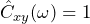Proof:

(2)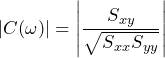(3)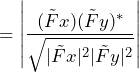(4)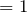Thus, the K=1 Welch estimate of coherence is always 1!  This is definitely an estimate; but the question is, “Is it a good estimate?”

To answer this question, let’s compute the true (non-estimated) coherence:

 (5)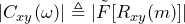by definition of coherence Cxy(ω) (6)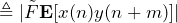by definition of cross-correlation Rxy (7)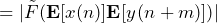because x[n] and y[n] are uncorrelated (8)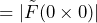because x[n] and y[n] have mean=0 (9)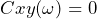because the Fourier transform of 0 is 0

So, we see that the true value of the coherence is Cxy(ω)= 0 (the smallest possible value), but the Welch estimate is Ĉxy(ω)= 1 (the largest possible value). It is likely that, in most cases, Welch will yield a very “good” estimate. However, as the above example demonstrates, this is not always the case and some caution is in order.